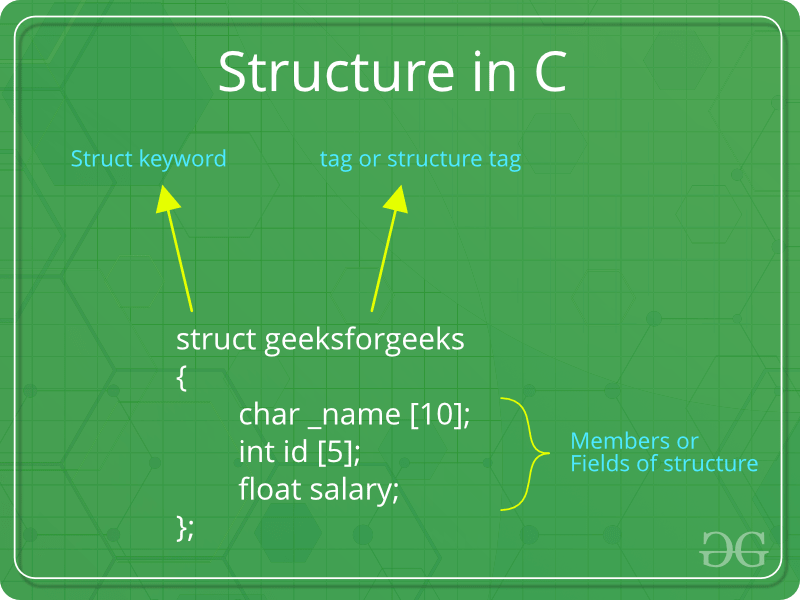# C - Arrow Operator

csk917work·2022년 4월 12일
0## Structure

• key word that create user defined data type in C/C++.
• creates a data type that can be used to group items of possibly different types into a single type.

### Structure Pointer

#include<stdio.h>

struct Point
{
int x, y;
};

int main()
{
// A valid initialization. member x gets value 1 and y
// gets value 2.
// The order of declaration is followed.
struct Point p1 = {1, 2};

// p2 is a pointer to structure p1
struct Point *p2 = &p1;

// Accessing structure members using structure pointer
printf("%d %d", p2->x, p2->y);
return 0;
}

// Output
// 1 2

### 정처기 예제

#include<stdio.h>

struct jsu
{
char nae;
int os, db, hab, hhab;
};

int main()
{
// A valid initialization. member os gets value 95 and db
// gets value 88.  The order of declaration is followed.
struct jsu st = {
{"데이터1", 95, 88},
{"데이터2", 84, 91},
{"데이터3", 86, 75}
};

struct jsu* p;

p = &st;

// 구조화하면 순차적으로 자동배치 된다.
// access field values using .

printf("CHAR Access \n");
printf("%s\n", (p) -> nae);
printf("%s\n", (p+1) -> nae);
printf("%s\n", (p+2) -> nae);

printf("INT Access \n");
printf("%d\n", (p) -> os);
printf("%d\n", (p+1) -> os);
printf("%d\n", (p+2) -> os);

printf("%d\n", (p) -> db);
printf("%d\n", (p) -> hab);
printf("%d\n", (p) -> hhab);

(p + 1)->hab = (p + 1)->os + (p + 2)->db;
(p + 1)->hhab = (p+1)->hab + p->os + p->db;
printf("\n");
printf("%d\n", (p+1)->hab + (p+1)->hhab);
}

// OUTPUT
/*
CHAR Access
데이터1
데이터2
데이터3
INT Access
95
84
86
88
0
0

501
*/

### Arrow operator in structure

// C program to show Arrow operator
// used in structure

#include <stdio.h>
#include <stdlib.h>

// Creating the structure
struct student {
char name;
int age;
float percentage;
};

// Creating the structure object
struct student* emp = NULL;

// Driver code
int main()
{
// Assigning memory to struct variable emp
emp = (struct student*)
malloc(sizeof(struct student));

// Assigning value to age variable
// of emp using arrow operator
emp->age = 18;

// Printing the assigned value to the variable
printf("%d", emp->age);

return 0;
}
// Output
// 18

### Arrow operator in unions

// C program to show Arrow operator
// used in structure

#include <stdio.h>
#include <stdlib.h>

// Creating the union
union student {
char name;
int age;
float percentage;
};

// Creating the union object
union student* emp = NULL;

// Driver code
int main()
{
// Assigning memory to struct variable emp
emp = (union student*)
malloc(sizeof(union student));

// Assigning value to age variable
// of emp using arrow operator
emp->age = 18;

// DIsplaying the assigned value to the variable
printf("%d", emp->age);
}
// Output
// 18

### 출처

https://www.geeksforgeeks.org/arrow-operator-in-c-c-with-examples/